Courses
Courses for Kids
Free study material
Offline Centres
More

# What Are Corresponding Sides?Last updated date: 01st Dec 2023
Total views: 98.7k
Views today: 2.98k## Introduction to Triangles

In Geometry, the three-sided polygon is a triangle that has three edges and three vertices. The most noteworthy property of a triangle is that the total sum of the interior angles of a triangle equals 180 degrees.

A triangle is a two-dimensional shape/ figure constituting three sides, three angles, and three vertices. The total sum of all internal angles of a triangle is invariably $180^{\circ}$ whether it is an isosceles, equilateral, or scalene triangle. The Sum of angles of the triangle is equal to 180 degrees. The external angles of a triangle always add up to 360 degrees.A fun cartoon of triangles

## What Is a True Triangle?

A true triangle is a shape that has three sides and three angles. The lengths of two of the sides must add up to a number greater than the third side, and the three angles must add up to $180^{\circ}$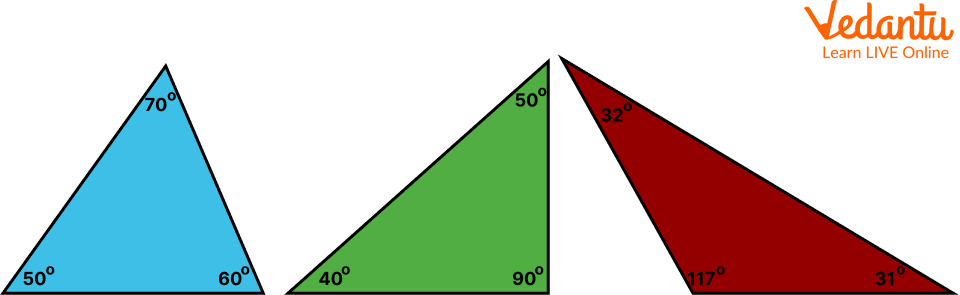The three angles of any above triangle will add up to $180^{\circ}$

## What Is the Formula for the Triangle?

The basic formula for the area of a triangle is equal to half the product of its base and height, i.e., $A = \frac{1}{2} \times b \times h$. This formula is applicable to all types of triangles, whether it is a scalene triangle, an isosceles triangle, or an equilateral triangle.

The angle sum property of a triangle states that the angles of a triangle always add up to $180^{\circ}$

$\angle \mathrm{A}+\angle \mathrm{B}+\angle \mathrm{C}=180^{\circ}$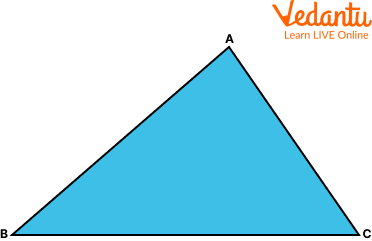Triangle ABC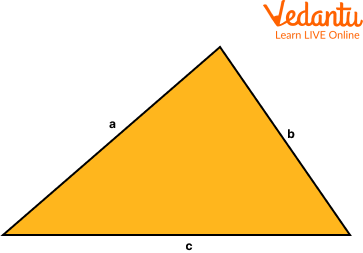Heron’s Formula

$S = \frac{a+b+c}{2}$

Here, S is the semi perimeter and a, b and c are the sides of the triangle.

$\text { Area of Triangle }=\sqrt{s(s-a)(s-b)(s-c)}$

## Corresponding Angles in Triangle and Its Sides

What is the meaning of the corresponding sides?

Corresponding sides and angles are a pair of matching angles or sides that are in the same spot in two different shapes.

Corresponding parts of congruent triangles or cpct tell us that corresponding sides and corresponding angles of the two triangles which are congruent are equal.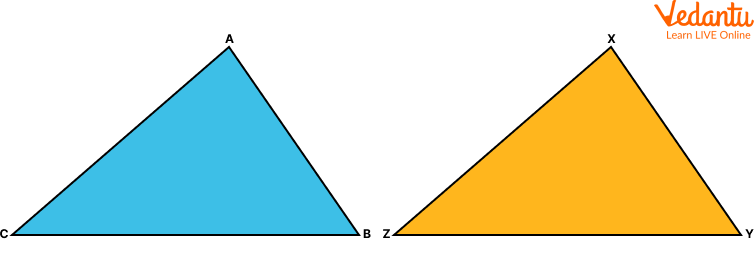Triangle ABC = Triangle XYZ

What is the example of the corresponding side?

For example, look at the above triangle ABC and XYZ.

Corresponding Angles

$\angle A \longleftrightarrow \angle X$

$\angle C \longleftrightarrow \angle Z$

$\angle B \longleftrightarrow \angle Y$

Corresponding sides

$\overline{A B} \longleftrightarrow \overline{XY}$

$\overline{B C} \longleftrightarrow \overline{YZ}$

$\overline{A C} \longleftrightarrow \overline{XZ}$

Note: Similar triangles have corresponding angles and corresponding sides.

## What is the Congruent Triangle?

Meaning of Congruent

If two figures can be placed precisely over each other, they are said to be 'congruent' figures. If you place one slice of bread over the other, you will find that both the slices are of equal shape and size. The term “congruent” means exactly equal shape and size.

Below are the congruent triangles examples.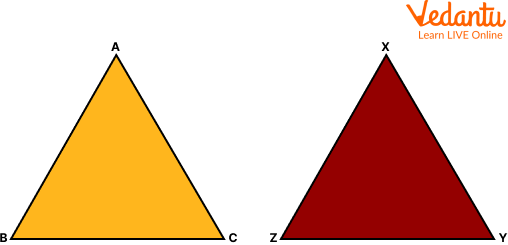Congruent triangles

How do you know if triangles are congruent?

If two sides and the included angle of one triangle are equal to the corresponding sides and angle of another triangle, the triangles are congruent.

## Congruence in Triangles

Congruence in two or more triangles depends on the measurements of their sides and angles. The three sides of a triangle determine its size and the three angles of a triangle determine its shape. Two triangles are said to be congruent if pairs of their corresponding sides and their corresponding angles are equal. They are of the same shape and size.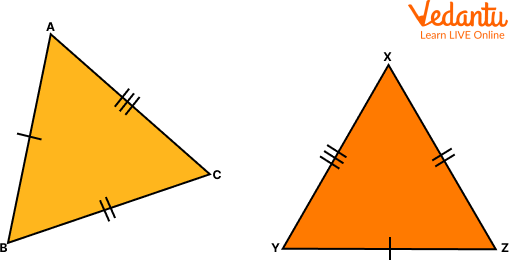Congruence in Triangles ABC and XYZ

## Naming Similar Triangles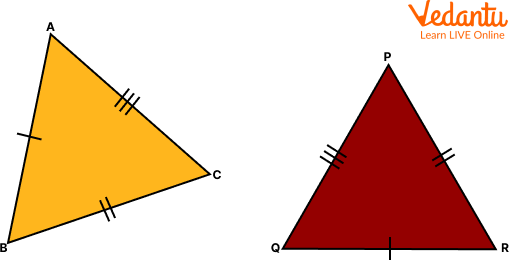Congruence in Triangles ABC and PQR

In the above figure, $\triangle \mathrm{ABC}$ and $\triangle \mathrm{PQR}$ are congruent triangles. This means,

Vertices: A and P, B and Q, and C and R are the same.

Sides: $A B=P Q, Q R=B C$ and $A C=P R$;

Angles: $\angle \mathrm{A}=\angle \mathrm{P}, \angle \mathrm{B}=\angle \mathrm{Q}$, and $\angle \mathrm{C}=\angle \mathrm{R}$.

Congruent triangles are triangles having corresponding sides and angles to be equal. The perimeter denotes congruence.

## Solved Questions

Q 1. In a $\triangle A B C$, if $A B=A C$ and $\angle B=70^{\circ}$, find $\angle A$.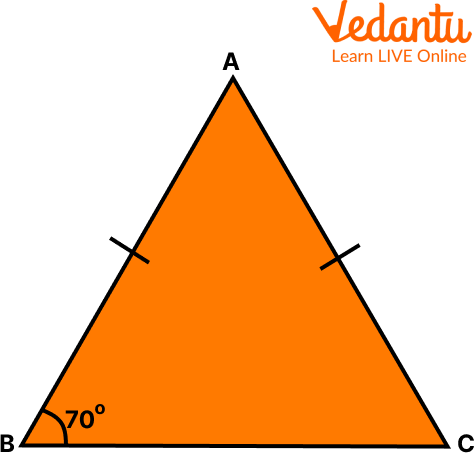Triangle ABC

Ans: Given: In a $\triangle \mathrm{ABC}, \mathrm{AB}=\mathrm{AC}$ and $\angle \mathrm{B}=70^{\circ}$

$\angle B=\angle C$ [Angles opposite to equal sides of a triangle are equal]

Therefore, $\angle \mathrm{B}=\angle \mathrm{C}=70^{\circ}$

Sum of angles in a triangle $=180^{\circ}$

$\angle \mathrm{A}+\angle \mathrm{B}+\angle \mathrm{C}=180^{\circ}$

$\angle \mathrm{A}+70^{\circ}+70^{\circ}=180^{\circ}$

$\angle \mathrm{A}=180^{\circ}-140^{\circ}$

$\angle \mathrm{A}=40^{\circ}$

Q 2. In a $\triangle \mathrm{ABC}$, if

$\mathrm{AB}^2=\mathrm{BC}^2+\mathrm{AC}^2$, then the right angle is at:

Ans: By Pythagoras theorem,

$(\text { hypotenuse })^2=(\text { perpendicular })^2+(\text { base })^2$

Clearly, $\mathrm{AB}$ is hypotenuse, $\mathrm{BC}$ and $\mathrm{AC}$ are either base or perpendicular.

Since, $A B$ is hypotenuse, therefore neither A nor $B$ is right angle.

Therefore, $C$ is the right angle.

## Practice Questions

Q 1. If $\triangle \mathrm{ABC}$ and $\triangle U Y T$ are similar triangles, then what sides/angles correspond with:Triangle ABC and UYT

(a) TU?

Ans: CA

(b) $\angle T U Y$?

Ans: $\angle C A B$

Q 2. Recognize congruent triangles

Decide whether this pair of triangles are congruent. If they are congruent, state why:Picture in reference to the question

(a) Check the corresponding angles and corresponding sides.?

Ans: Triangles have sides of 6.3cm,8.1cm, and 10.2cm.

(b) Decide if the shapes are congruent or not.

Ans: The triangles are the same shape and the same size – they are congruent.

## Summary

There are six types of triangles– Isosceles, Scalene, Equilateral, Oblique, Acute, and Right. Based on the type according to internal angles, there are three types – Equilateral, Scalene, and Isosceles. Whereas, the kinds of a triangle are classified according to the length of their sides which are Right, Acute, and Oblique.

The Properties of a Triangle Are:

1. A triangle contains three sides, three angles, and three vertices.

2. The totality of all interior angles of a triangle is equal to $180^{\circ}$. This is known as the angle sum of a triangle.

3. The total sum of the length of any two sides of a triangle is greater than the length of its third side.

4. The side which is opposite the largest angle of a triangle is its largest side.

## FAQs on What Are Corresponding Sides?

1. What is a Scalene triangle?

A triangle that has all three sides of different lengths is a scalene triangle.

2. What is an Isosceles triangle?

A triangle that has two sides of the same length and the third side of a different length is an isosceles triangle.

3. What is an equilateral triangle?

A triangle that has all three sides of the same length is an equilateral triangle.